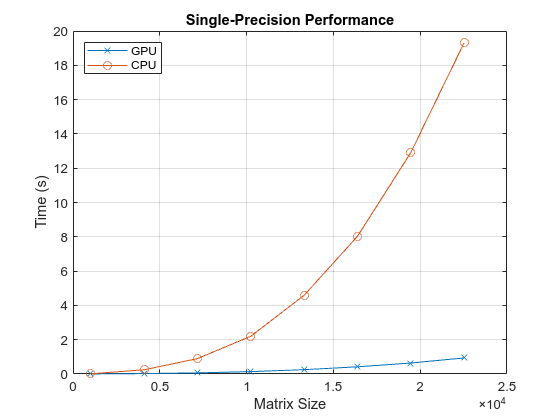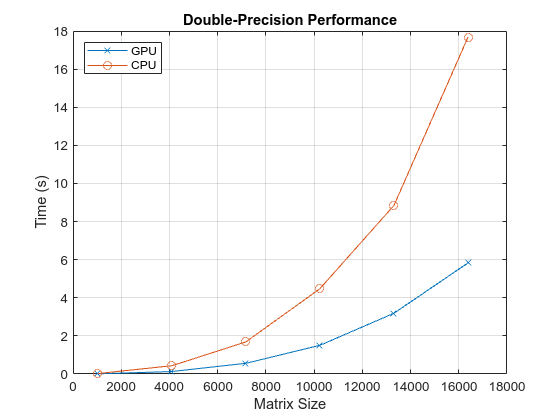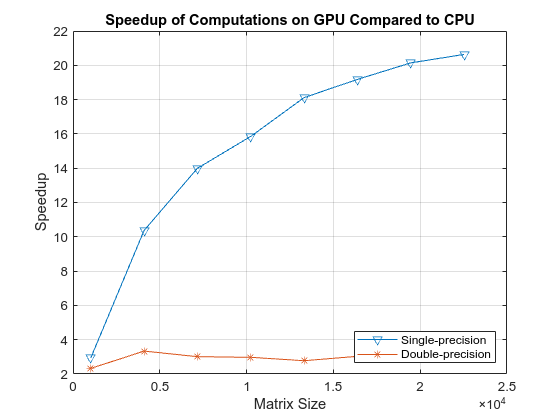# Benchmark Solving a Linear System by Using GPU Coder

This example shows how to benchmark solving a linear system by generating CUDA® code. Use matrix left division, also known as `mldivide` or the backslash operator (\), to solve the system of linear equations `A*x = b` for `x` (that is, compute `x = A\b`).

### Third-Party Prerequisites

#### Required

This example generates CUDA MEX and has the following third-party requirements.

• CUDA enabled NVIDIA® GPU and compatible driver.

#### Optional

For non-MEX builds such as static, dynamic libraries or executables, this example has the following additional requirements.

### Verify GPU Environment

To verify that the compilers and libraries necessary for running this example are set up correctly, use the `coder.checkGpuInstall` function.

```envCfg = coder.gpuEnvConfig('host'); envCfg.BasicCodegen = 1; envCfg.Quiet = 1; coder.checkGpuInstall(envCfg);```

### Determine the Maximum Data Size

Choose the appropriate matrix size for the computations by specifying the amount of system memory in GB available to the CPU and the GPU. The default value is based only on the amount of memory available on the GPU. You can specify a value that is appropriate for your system.

```g = gpuDevice; maxMemory = 0.1*g.AvailableMemory/1024^3;```

#### Note:

This example uses cuSOLVER libararies that have significant GPU memory requirements for creating workspaces. If you run into CUDA out-of-memory errors, reduce the `maxMemory` or the matrix step sizes in `sizeSingle` and `sizeDouble`.

### The Benchmarking Function

This example benchmarks matrix left division (\) including the cost of transferring data between the CPU and GPU, to get a clear view of the total application time when using GPU Coder™. The application time profiling must not include the time to create sample input data. The `genData.m` function separates generation of test data from the entry-point function that solves the linear system.

`type getData.m`
```function [A, b] = getData(n, clz) % Copyright 2017-2022 The MathWorks, Inc. fprintf('Creating a matrix of size %d-by-%d.\n', n, n); A = rand(n, n, clz) + 100*eye(n, n, clz); b = rand(n, 1, clz); end ```

### The Backslash Entry-Point Function

The `backslash.m` entry-point function encapsulates the (\) operation for which you want to generate code.

`type backslash.m`
```function [x] = backslash(A,b) %#codegen % Copyright 2017-2022 The MathWorks, Inc. coder.gpu.kernelfun(); x = A\b; end ```

### Generate the GPU Code

Create a function to generate the GPU MEX function based on the particular input data size.

`type genGpuCode.m`
```function [] = genGpuCode(A, b) % Copyright 2017-2022 The MathWorks, Inc. cfg = coder.gpuConfig('mex'); evalc('codegen -config cfg -args {A,b} backslash'); end ```

### Choose a Problem Size

The performance of the parallel algorithms that solve a linear system depends greatly on the matrix size. This example compares the performance of the algorithm for different matrix sizes (multiples of 1024).

```sizeLimit = inf; if ispc sizeLimit = double(intmax('int32')); end maxSizeSingle = min(floor(sqrt(maxMemory*1024^3/4)),floor(sqrt(sizeLimit/4))); maxSizeDouble = min(floor(sqrt(maxMemory*1024^3/8)),floor(sqrt(sizeLimit/8))); step = 1024; if maxSizeDouble/step >= 10 step = step*floor(maxSizeDouble/(5*step)); end sizeSingle = 1024:step:maxSizeSingle; sizeDouble = 1024:step:maxSizeDouble; numReps = 5;```

### Compare Performance: Speedup

Use the total elapsed time as a measure of performance because that enables you to compare the performance of the algorithm for different matrix sizes. Given a matrix size, the benchmarking function creates the matrix `A` and the right-side `b` once, and then solves `A\b` a few times to get an accurate measure of the time it takes.

`type benchFcnMat.m`
```function time = benchFcnMat(A, b, reps) % Copyright 2017-2022 The MathWorks, Inc. time = inf; % Solve the linear system a few times and take the best run for itr = 1:reps tic; matX = backslash(A, b); tcurr = toc; time = min(tcurr, time); end end ```

Create a different function for GPU code execution that invokes the generated GPU MEX function.

`type benchFcnGpu.m`
```function time = benchFcnGpu(A, b, reps) % Copyright 2017-2022 The MathWorks, Inc. time = inf; gpuX = backslash_mex(A, b); for itr = 1:reps tic; gpuX = backslash_mex(A, b); tcurr = toc; time = min(tcurr, time); end end ```

### Execute the Benchmarks

When you execute the benchmarks, the computations can take a long time to complete. Print some intermediate status information as you complete the benchmarking for each matrix size. Encapsulate the loop over all the matrix sizes in a function to benchmark single- and double-precision computations.

Actual execution times can vary across different hardware configurations. This benchmarking was done by using MATLAB R2022a on a machine with a 6 core, 3.5GHz Intel® Xeon® CPU and an NVIDIA TITAN Xp GPU.

`type executeBenchmarks.m`
```function [timeCPU, timeGPU] = executeBenchmarks(clz, sizes, reps) % Copyright 2017-2022 The MathWorks, Inc. fprintf(['Starting benchmarks with %d different %s-precision ' ... 'matrices of sizes\nranging from %d-by-%d to %d-by-%d.\n'], ... length(sizes), clz, sizes(1), sizes(1), sizes(end), ... sizes(end)); timeGPU = zeros(size(sizes)); timeCPU = zeros(size(sizes)); for i = 1:length(sizes) n = sizes(i); fprintf('Size : %d\n', n); [A, b] = getData(n, clz); genGpuCode(A, b); timeCPU(i) = benchFcnMat(A, b, reps); fprintf('Time on CPU: %f sec\n', timeCPU(i)); timeGPU(i) = benchFcnGpu(A, b, reps); fprintf('Time on GPU: %f sec\n', timeGPU(i)); fprintf('\n'); end end ```

Execute the benchmarks in single and double precision.

`[cpu, gpu] = executeBenchmarks('single', sizeSingle, numReps);`
```Starting benchmarks with 9 different single-precision matrices of sizes ranging from 1024-by-1024 to 17408-by-17408. Size : 1024 Creating a matrix of size 1024-by-1024. Time on CPU: 0.012281 sec Time on GPU: 0.008329 sec Size : 3072 Creating a matrix of size 3072-by-3072. Time on CPU: 0.115839 sec Time on GPU: 0.035071 sec Size : 5120 Creating a matrix of size 5120-by-5120. Time on CPU: 0.380651 sec Time on GPU: 0.074228 sec Size : 7168 Creating a matrix of size 7168-by-7168. Time on CPU: 0.867239 sec Time on GPU: 0.127977 sec Size : 9216 Creating a matrix of size 9216-by-9216. Time on CPU: 1.677065 sec Time on GPU: 0.205344 sec Size : 11264 Creating a matrix of size 11264-by-11264. Time on CPU: 2.911081 sec Time on GPU: 0.306867 sec Size : 13312 Creating a matrix of size 13312-by-13312. Time on CPU: 4.684644 sec Time on GPU: 0.440095 sec Size : 15360 Creating a matrix of size 15360-by-15360. Time on CPU: 6.950956 sec Time on GPU: 0.608897 sec Size : 17408 Creating a matrix of size 17408-by-17408. Time on CPU: 9.833478 sec Time on GPU: 0.802604 sec ```
```results.sizeSingle = sizeSingle; results.timeSingleCPU = cpu; results.timeSingleGPU = gpu; [cpu, gpu] = executeBenchmarks('double', sizeDouble, numReps);```
```Starting benchmarks with 6 different double-precision matrices of sizes ranging from 1024-by-1024 to 11264-by-11264. Size : 1024 Creating a matrix of size 1024-by-1024. Time on CPU: 0.021463 sec Time on GPU: 0.010796 sec Size : 3072 Creating a matrix of size 3072-by-3072. Time on CPU: 0.213805 sec Time on GPU: 0.093114 sec Size : 5120 Creating a matrix of size 5120-by-5120. Time on CPU: 0.689023 sec Time on GPU: 0.323026 sec Size : 7168 Creating a matrix of size 7168-by-7168. Time on CPU: 1.687437 sec Time on GPU: 0.775834 sec Size : 9216 Creating a matrix of size 9216-by-9216. Time on CPU: 3.521580 sec Time on GPU: 1.539601 sec Size : 11264 Creating a matrix of size 11264-by-11264. Time on CPU: 6.075310 sec Time on GPU: 2.694465 sec ```
```results.sizeDouble = sizeDouble; results.timeDoubleCPU = cpu; results.timeDoubleGPU = gpu;```

### Plot the Performance

Plot the results and compare the performance on the CPU and the GPU for single and double precision.

First, look at the performance of the backslash operator in single precision.

```fig = figure; ax = axes('parent', fig); plot(ax, results.sizeSingle, results.timeSingleGPU, '-x', ... results.sizeSingle, results.timeSingleCPU, '-o') grid on; legend('GPU', 'CPU', 'Location', 'NorthWest'); title(ax, 'Single-Precision Performance') ylabel(ax, 'Time (s)'); xlabel(ax, 'Matrix Size');````drawnow;`

Now, look at the performance of the backslash operator in double precision.

```fig = figure; ax = axes('parent', fig); plot(ax, results.sizeDouble, results.timeDoubleGPU, '-x', ... results.sizeDouble, results.timeDoubleCPU, '-o') legend('GPU', 'CPU', 'Location', 'NorthWest'); grid on; title(ax, 'Double-Precision Performance') ylabel(ax, 'Time (s)'); xlabel(ax, 'Matrix Size'); drawnow;```Finally, look at the speedup of the backslash operator when comparing the GPU to the CPU.

```speedupDouble = results.timeDoubleCPU./results.timeDoubleGPU; speedupSingle = results.timeSingleCPU./results.timeSingleGPU; fig = figure; ax = axes('parent', fig); plot(ax, results.sizeSingle, speedupSingle, '-v', ... results.sizeDouble, speedupDouble, '-*') grid on; legend('Single-precision', 'Double-precision', 'Location', 'SouthEast'); title(ax, 'Speedup of Computations on GPU Compared to CPU'); ylabel(ax, 'Speedup'); xlabel(ax, 'Matrix Size'); drawnow;```